# SSC CGL 2018 Practice Test Papers | Quantitative Aptitude (Day-46)

Dear Aspirants, Here we have given the Important SSC CGL Exam 2018 Practice Test Papers. Candidates those who are preparing for SSC CGL 2018 can practice these questions to get more confidence to Crack SSC CGL 2018 Examination.

[WpProQuiz 3446]

Click “Start Quiz” to attend these Questions and view Explanation

1) If a/b=c/d then what is the value of (ab+cd)2?

a) (a+c) 2 (b-d) 2

b) (b2+d2) (a2 + d2)

c) (a+c)2(b+d)2

d) a2b2+c2d2

2)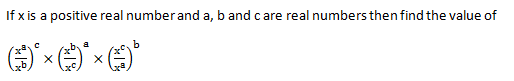a) 2

b) 1

c) 0

d) -2

3)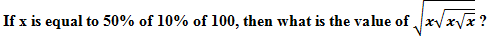a) 57/8

b) 63/7

c) 91/2

d) None of the above

4) The first and last terms of AP are 75 and 171. If there are 9 terms in this sequence, Find the sum of third and fifth terms of the sequence.

a) 122

b) 222

c) 230

d) 150

5) There is a regular polygon and if we reduce it’s no of sides by 2 then its number of diagonals reduced by 19 then how many sides does it have initially?

a) 10

b) 11

c) 12

d) 9

6) If cosec y+ cot y=2+ √3 Find the value of tan 3y/2.

a) 0

b) -1

c) -√3

d) 1

7) P and Q completed a job by working alternate days. They took a total of ten days to complete it. Find the time required to complete the work if they work together?

a) 4

b) 5

c) 6

d) 3.2

8) In a class, average age of 30 students is 18 years. If the ages of 2 more students are taken into consideration, then the average of all the students gets increase by 1. Find the average of the ages of those 2 students.

a) 33

b) 32

c) 34

d) 36

9) A certain number of people decided to meet in XYZ College in an alumni meet and decided to construct a block for XYZ College. But every month four people step out of this plan. Due to this the task is completed in half more year instead of one year. Then how many people were originally involved in this plan?

a) 100

b) 124

c) 102

d) 104

10) Find the decreased percentage of volume of the circular cylinder which radius and height decreased by 20%.

a) 51.75

b) 48.8

c) 38.8

d) 62.3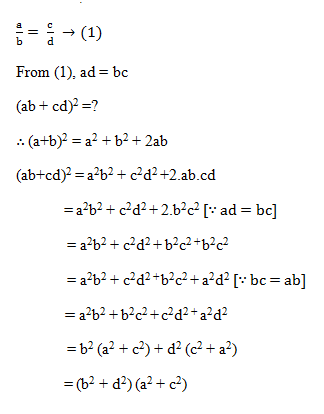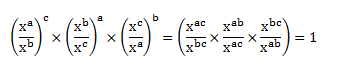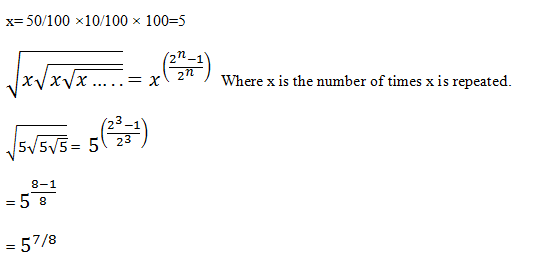We know that,

Last term in an arithmetic progression = a+ (n-1) d = 171 [a = first term, n = no. of terms]

75+ (9-1) d = 171

d (Common difference) = 12.

So 3rd term is 75 + 2×12 = 99 and 5th term = 75 + 4×12 = 123.

So the required answer is 99 + 123 = 222.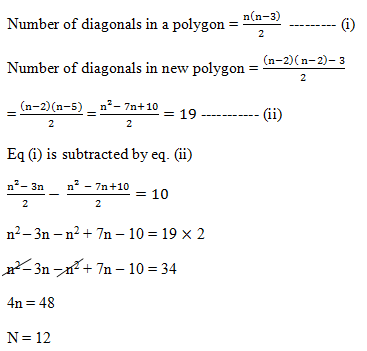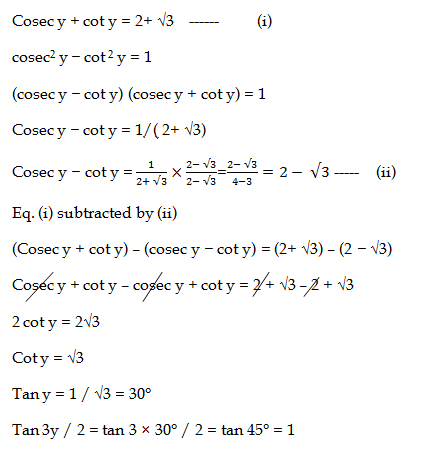Work done by (P+Q) together on a single day = Work done by P on first day+ Work done by Q on Second day

So the time required to complete the work (P+Q) together = 10/2= 5 days

Average of 30 students = 18

Total ages of Students = 18 x30 =540

Average of 32 students = 19

Total ages 32 Students = 19 x32 =608

So total ages of those 2 students = 608 – 540 = 68

Average = 68/2 = 34

Let the total number of people = x

Work is completed in one and a half year = 18 months

Then,

12x = (x+ (x – 4) + (x – 8) + (x – 12) + (x – 16) + (x – 20) + … 17 times)

12x = 18x – 4 (1 + 2 + 3 + … + 17)

6x = (4 ×17×18)/2
x = 102

Volume of cylinder= π r2 h

Let the radius and height = 10 cm

So area = π×10×10×10 =1000 π cm2

After decrease

New radius = 10 – 20× 10 /100 = 8 cm

New height = 10-20× 10 /100 = 8 cm

New volume = π×8×8×8 = 512 π cm2

Decreased volume = 488 π cm2

Percentage decrease = 488π× 100 /1000π = 48.8%